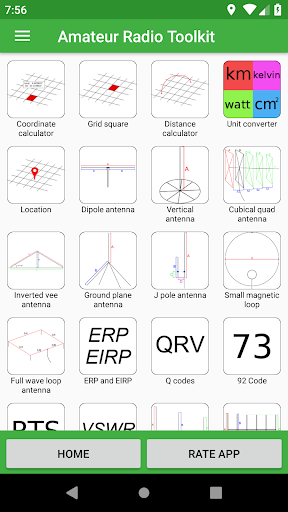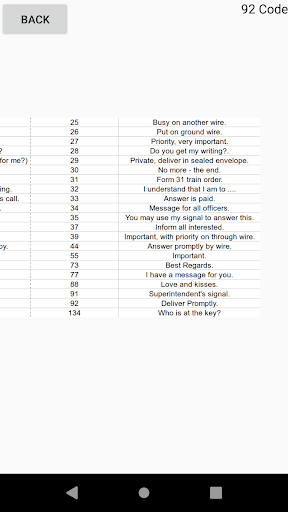Tools:
Q code – A list of common Q codes
92 Code – A list of common 92 codes
RST code – A list of RST codes
Distance calculator – Find the distance between two Maidenhead squares
Unit converter – Convert between units
Dipole antenna – Calculate the optimal lengths of a dipole antenna for a given frequency
Vertical antenna – Calculate the optimal lengths of a vertical antenna for a given frequency
Cubical quad antenna – Calculate the optimal lengths of a cubical quad antenna for a given frequency
Inverted Vee antenna – Calculate the optimal lengths of an inverted vee antenna for a given frequency
Ground plane antenna – Calculate the optimal lengths of a ground plane antenna for a given frequency
J pole antenna – Calculate the optimal lengths of a J pole antenna for a given frequency
VSWR – Convert between VSWR, Refection coefficient, return loss and mismatch loss
Three element Yagi antenna – Calculate the optimal lengths of a three-element Yagi antenna for a given frequency
Seven-element Yagi antenna – Calculate the optimal lengths of a seven element Yagi antenna for a given frequency
Wavelength frequency converter – Convert between wavelength and frequency
Air core inductor – Calculate the inductance or lengths of an air core inductor
Low pass filter – Calculate the units of an RC or RL LPF circuit
High pass filter – Calculate the units of an RC or RL HPF circuit
Ohm’s law – Convert between voltage, current, resistance and power by entering two
Reactance – Calculate the reactance of a capacitor or inductor
Decibel calculator – Convert between decibel values or calculate dB by entering the power or voltage
Voltage divider – Calculate the output voltage or resistance of one resistor
Resistor Color codes – Find the resistance of a resistor by entering the colors of the bands
Resistors in parallel – Calculate the resistance of resistors in parallel
Resistors in series – Calculate the resistance of resistors in series
Capacitors in parallel – Calculate the capacitance of capacitors in parallel
Capacitors in series – Calculate the capacitance of capacitors in series
Coaxial cable – Calculate the properties of a coaxial cable
Morse code
CW abbreviations
NATO phonetic alphabet

You can upgrade to the Pro version to remove all ads and get the following features:

Half wave coax balun – Calculate the optimal length of a half wave coax balun for a given frequency
Narrowband balun – Calculate the inductance and capacitance of a narrowband balun
Small magnetic loop – Calculate the optimal lengths of a small magnetic loop for a given frequency
Full wave loop antenna – Calculate the optimal lengths of a full wave loop antenna for a given frequency
ERP and EIRP – Calculate and convert ERP and EIRP
RMS voltage – Calculate the RMS voltage
Pi attenuator – Calculate the values of the resistors by entering the attenuation and impedance
T attenuator – Calculate the values of the resistors by entering the attenuation and impedance
Inductors in series – Calculate the inductance of inductors in series
Inductors in parallel – Calculate the inductance of inductors in parallel
Doppler shift – Calculate the Doppler shift
Bug fixes
New features:
Q codes
92 Code
RST Code
Morse code
CW abbreviations
Nato phonetic alphabet